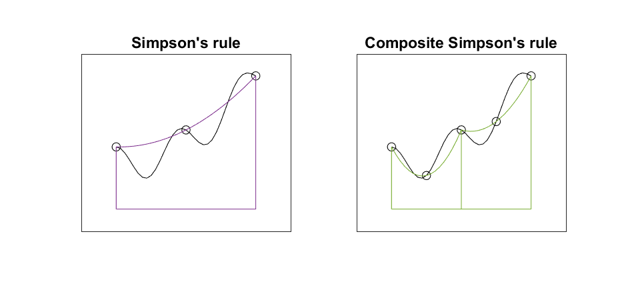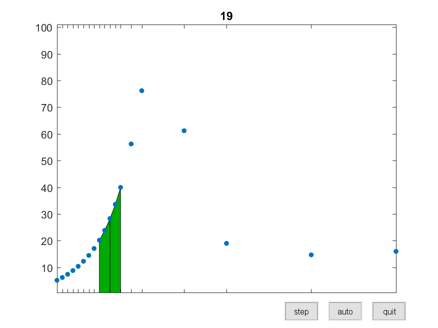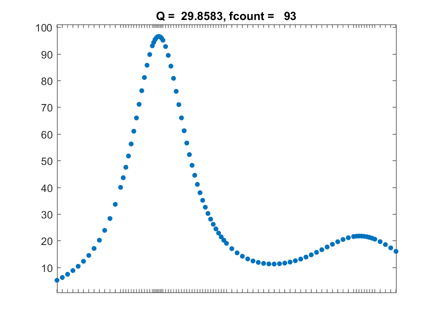# Modernization of Numerical Integration, From Quad to Integral

The MATLAB functions for the numerical evaluation of integrals has evolved from quad, through quadl and quadgk, to today's integral.

### Contents

Several years ago I regarded myself as an expert on numerical methods for approximating integrals. But then I stopped paying attention for a while. So this is an opportunity to get reacquainted. The task involves a real-valued function $f(x)$ of a real variable $x$, defined on an interval $a \le x \le b$. We seek to compute the value of the definite integral, $$\int_a^b \ f(x) \ dx$$ I wrote a chapter on the subject in my textbook Numerical Computing with MATLAB. Here is a link to that chapter. The chapter is titled "Quadrature". One of the first things I have to do in that chapter is explain that quadrature is an old-fashioned term derived from the notion that the value of a definite integral can be approximated by plotting the function on graph paper and then counting the number of little squares that fall underneath the graph. Here is a coarse picture.For most of the time since the beginning of MATLAB, up until when I wrote the chapter on quadrature twelve years ago, the MATLAB function for evaluating definite integrals was one named quad. It was based on adaptive Simpson's method, pioneered by my grad school buddy Bill McKeeman to show off recursion in Algol. These two figures illustrate the basis for adaptive Simpson's method.The algorithm works recursively on subintervals of the original interval [a,b]. If the values obtained from the 3-point Simpson's rule and the 5-point composite Simpson's rule agree to within a specified tolerance (which they clearly do not for these figures), then an extrapolation taken from the two values is accepted as the value for the integral over the subinterval. If not, the algorithm is recursively applied to the two halves of the subinterval.

I have used a function named humps to demonstrate numerical integration for a long time. It has a strong peak at x = 0.3 and a milder peak at x = 0.9. It has found its way into the MATLAB demos directory, so you have it on your own machine. A graph appears below. $$h(x) = \frac{1}{(x-0.3)^2+.01} + \frac{1}{(x-0.9)^2+.04} - 6$$ An interactive graphical app named quadgui is included with the programs for Numerical Computing with MATLAB, available from MATLAB Central File Exchange. It shows quad in action. Here is a snapshot of quad part way through computing the integral of humps over the interval [0,1]. Integration over the interval to the left of 1/4 is finished. The integration over the subinterval [1/4,3/8] has just been successfully completed. A few samples to the right of 3/8 were computed during the initialization. The integrand has been evaluated 19 times.Here is the final result. The default tolerance for quad is $10^{-6}$ and the value of the integral obtained to six significant digits is 29.8583. The integrand has been evaluated 93 times. Most of those evaluations took place near the peaks where the humps varies rapidly. This indicates that the adaptivity works as expected.Humps is a rational function. It is possible to use the Symbolic Toolbox to obtain the indefinite and then the definite integral analytically.
   syms x
h = 1/((x-3/10)^2+1/100) + 1/((x-9/10)^2+4/100) - 6
I = int(h,x)
D = subs(I,1)-subs(I,0)
format long
Q = double(D)

h =
1/((x - 9/10)^2 + 1/25) + 1/((x - 3/10)^2 + 1/100) - 6
I =
10*atan(10*x - 3) - 6*x + 5*atan(5*x - 9/2)
D =
5*atan(1/2) + 10*atan(3) + 10*atan(7) + 5*atan(9/2) - 6
Q =
29.858325395498674

This confirms the six figure value shown by quadqui

About the time in 2004 that I was writing the Quadrature chapter for NCM, the quadl function showed up. This uses the Lobatto rule. $$\int_{-1}^1 \ f(x) \ dx \ \approx \ w_1 f(-1) + w_2 f(-x_1) + w_2 f(x_1) + w_1 f(1)$$ with $x_1 = 1/\sqrt{5}, \ w_1 = 1/6, \ w_2 = 5/6$. Quadl also employs the associated Kronrod rule, which provides 13 additional irrational abscissa and weights. The Lobatto-Kronrod rule is much higher order and consequently more accurate than Simpson's rule. As a result quadl is usually faster and more accurate than quad. But I just devoted a couple of exercises in the book to quadl. And I think it never became very popular.

In the mathematical software business when we were developing libraries using Fortran or C, we evaluated the cost of numerical quadrature by counting the number of function evaluations. In 2006 Larry Shampine, the SMU Professor who is a consultant to MathWorks and principal author of our ODE Suite, published the paper referenced below where he changed the ground rules for quadrature functions in the MATLAB environment. Since MATLAB function evaluations are vectorized, we should count the number of vectorized evaluations, not the number of individual evaluations. This is a better predictor of execution time. Shampine's paper includes a MATLAB function that he calls quadva, where va stands for "vectorized adaptive". His code uses a 15-point Gauss-Kronrod formula. The Gauss formula is not only higher order than the Lobatto formula with the same number of points, it has the additional advantage that it does not require evaluating the integrand at the end points of an interval. This makes it possible to treat infinite intervals and singularites at the end points. When we added Shampine's quadva to MATLAB, we called it quadgk, for "Gauss-Kronrod".

#### Comparison on humps.

Let's instrument humps to investigate the behavior of our quadrature routines on just one simple test case. The functions quad and quadl call the integrand once with a vector argument to initialize the integration and then call it more times with a shorter vector argument during the adaptive process. This is such an easy problem that quadgk gets all the information that it needs during the initialization. In the following table, "init" is the length of the vector in the initialization call, "kount" is the number of times that humps is called after the initialization, "length" is the length of the vector argument in those calls, "err" is the error in the final result, and "time" is the execution time in milliseconds. $$\begin{array}{lrrrrr} & \mbox{init} & \mbox{kount} & \mbox{length} & \mbox{err\ \ \ \ } & \mbox{time}\\ \mbox{quad} & 7 & 69 & 2 & 7.3 \cdot 10^{-7} & 0.87 \\ \mbox{quadl} & 13 & 37 & 5 & 1.8 \cdot 10^{-10} & 0.78 \\ \mbox{quadgk} & 150 & 0 & - & 2.5 \cdot 10^{-14} & 0.34 \end{array}$$ We see that the three functions perform very differently in this one experiment. The oldest function, quad, actually requires the fewest total function evaluations, if we count them in the traditional scalar sense, but it is the least accurate and the slowest. The newest function, quadgk, requires only one vectorized function evaluation, and is the fastest and the most accurate.

In 2012, with release 2012a, we came to the realization that most new MATLAB users who wanted to evaluate an integral had a hard time finding the function named "quadgk" that does the job. For all these years, we numerical analysts have been explaining to the rest of the world our quaint term quadrature. If that weren't enough, we have to tack on initials of mathematicians. So, we introduced integral. Actually quadgk is still available. Integral is just an easier to find and easier to use version of quadgk.

#### Reference

L.F. Shampine, "Vectorized Adaptive Quadrature in MATLAB, Journal of Computational and Applied Mathematics 211 (2006), pp.131–140, available at <http://faculty.smu.edu/shampine/rev2vadapt.pdf>

Published with MATLAB® R2016a

|

### コメント

コメントを残すには、ここ をクリックして MathWorks アカウントにサインインするか新しい MathWorks アカウントを作成します。